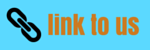# Practice questions for Algebraic Expressions and Identities Class 8 Maths Chapter 9

In this page we have Practice questions for Algebraic Expressions and Identities Class 8 Maths Chapter 9 . Hope you like them and do not forget to like , social share and comment at the end of the page.
Question 1
Identify the terms, their coefficients for each of the following expressions.
(i) xyz2 + 3xy
(ii) 1 - x -2 x2
(iii) 4p2q2 – 4p2q2r2 + r2
(iv) 4 – xy + yz – xz
(v) (x/4) –(y/5) -y
(vi) 1.3a – 2.6ab + 1.5b
Question 2
Classify the following polynomials as monomials, binomials, trinomials. Which polynomials do not fit in any of these three categories?
x2 + y2
1000-x
x + x2 + x3 + x4 + x5
8 - y +-5x
2y – 3y2
2y – 3y + 4y3
5x – 8y + 3xy
4 – 15z2
ab + bc + cd + da+ 2ab
pqr+2pq+5pqr
p2q + pq2
2p + 2q+1
Question 3
(i) ab – bc+ ac, bc – ca+ ab, ca – ab-2bc
(ii) p – q + pq, q – r + qr, r – p + pr , p+q+r
(iii) 2p2q2 – 3pq + 4, 5 + 7pq – 3p2q2 ,4p2q2 + 10pq
(iv) a2 + b2, b2 + c2, c2 + a2, 2ab + 2bc + 2ac
Question 4.
(a) Subtract 8a – 7ab + 3b - 20 from 20a – 9ab + 5b – 20
(b) Subtract 3pq + 5qr – 7pr+1 from -4pq +2qr – 2pr + 5pqr+1
(c) Subtract 4p2q – 4pq - 5pq2 – 8p + 7q – 18
from 18 – 3p – 11q + 5pq – 2pq2 + 5p2q

Question 5
What are the coefficient of each term in the below expression?
4p2q2 + 4p2q2r2 - r2 +5
a)  4,4,-1,5
b)  4,4,1,5
c) 4,4r2 ,-r2, 5
d) None of these
Question 6
The product of a monomial and trinomial will be a
a)  monomial
b)  trinomial
c) binomial
d) None of these
Question 7
The exponents of a variable term in the polynomial is a
a) integers
b)  negative integers
c) positive integers
d) non -negative integers

Question 8
The expression pqr +rqp+qpr is a
a) Monomial
b)  trinomial
c) binomial
d) none of these